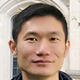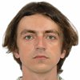## Joint Chinese-Russian Mathematical Online Colloquium

##### 11:00 (GMT+3)Yifeng Liu Zhejiang University Bio: Yifeng Liu is a Professor at the Institute for Advanced Study in Mathematics, Zhejiang University. His primary areas of interest span algebraic geometry, automorphic representations, and number theory. He obtained his B.S. degree from Peking University in 2007 and PhD from Columbia University in 2012. He was a professor at Yale before joining Zhejiang University in 2021. Yifeng Liu received a Sloan Research Fellowship in 2017 and was awarded the SASTRA-Ramanujan Prize in 2018 for his contribution to number theory and arithmetic geometry.

### Congruence of modular forms and arithmetic of Shimura varieties.

The congruence of modular forms is an important phenomenon in the arithmetic study of modular forms, or more generally, automorphic forms. For classical modular forms, many results have been obtained by Serre, Ribet, et al, for more than thirty years. In particular, Ribet used the arithmetic geometry of modular curves to find such congruence relation, also known as level raising. We recall as follows: Fix a prime l; consider a weight-2 level-N newform f satisfying the mod l level-raising condition at a prime p coprime to Nl. Ribet proved that the first Galois cohomology of the mod l Galois representation of Q_p associated with f can be realized as the Abel-Jacobi image of the supersingular locus of the level-N modular curve over F_p.

In ongoing joint work with Yichao Tian (MCM) and Liang Xiao (PKU), we generalize this phenomenon to higher-dimensional unitary Shimura varieties at inert places (which remains a conjecture in general), and its relation with a certain Ihara type lemma for such varieties. In the talk, I will explain cases for which we have confirmed such conjecture; and if time permits, we will mention its number-theoretical implications.

##### 12:00 (GMT+3)Anton Fonarev Steklov Mathematical Institute of RAS Bio: Anton Fonarev is a Senior Scientific Researcher in the Department of Algebraic Geometry of Steklov Mathematical Institute of RAS. He graduated from the Faculty of Mechanics and Mathematics of Lomonosov Moscow State University in 2011 and obtained his PhD at Steklov Mathematical Institute in 2014. Then he was a scientific researcher in the Laboratory of Algebraic Geometry and in the Laboratory of Mirror Symmetry and Automorphic Forms of Higher School of Economics in Moscow. His research interests include algebraic geometry and homological algebra.

### 45 years of derived categories of rational homogeneous varieties.

In 1978, Beilinson published a celebrated paper in which he showed that the bounded derived category of a projective space admits a full exceptional collection. Since then mathematicians have been trying to prove that the same holds for all rational homogeneous varieties. We will give a gentle introduction to this problem and give an overview of the progress that has been made.

The meeting will be held in the form of a webinar on the Zoom platform.

Pre-registration for the event is not required.